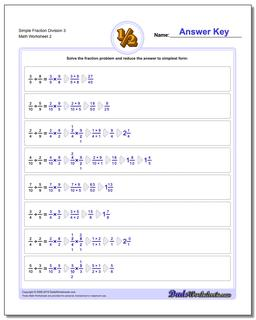# Math Worksheets: Fraction Division: Fraction Division: Simple Fraction Division 3 (Second Worksheet)## Simple Fraction Division 3 (Second Worksheet)

PropertyValue
DescriptionSimple Fraction Division 3: Worksheets for basic division of simple fractions, no mixed fractions or cross-cancelling (Second Worksheet)
Resource TypeWorksheet